Dishashree26 Gupta — Published On April 10, 2017 and Last Modified On June 22nd, 2022

## Introduction

Probability forms the backbone of many important data science concepts from inferential statistics to Bayesian networks. It would not be wrong to say that the journey of mastering statistics begins with probability. This skilltest was conducted to help you identify your skill level in probability.

A total of 1249 people registered for this skill test. The test was designed to test the conceptual knowledge of probability. If you are one of those who missed out on this skill test, here are the questions and solutions. You missed on the real time test, but can read this article to find out how you could have answered correctly.

Here are the leaderboard ranking for all the participants.

Are you preparing for your next data science interview? Then look no further! Check out the comprehensive ‘Ace Data Science Interviews‘ course which encompasses hundreds of questions like these along with plenty of videos, support and resources. And if you’re looking to brush up your probability sills even more, we have covered it comprehensively in the ‘Introduction to Data Science‘ course!

## Overall Scores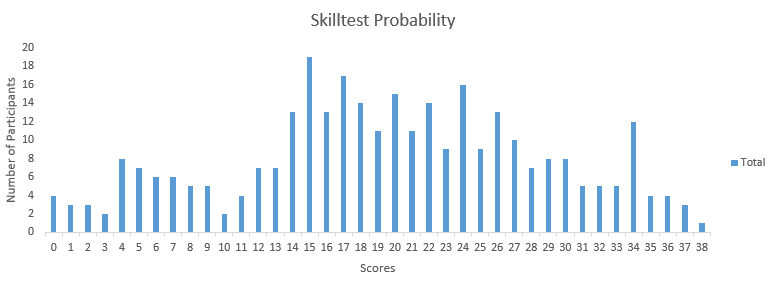You can access the final scores here. More than 300 people participated in the skill test and the highest score obtained was 38. Here are a few statistics about the distribution.

Mean Score: 19.56

Median Score: 20

Mode Score: 15

This was also the first test where some one scored as high as 38! The community is getting serious about DataFest

## Useful Resources

Basics of Probability for Data Science explained with examples

Introduction to Conditional Probability and Bayes theorem for data science professionals

1) Let A and B be events on the same sample space, with P (A) = 0.6 and P (B) = 0.7. Can these two events be disjoint?

A) Yes

B) No

2) Alice has 2 kids and one of them is a girl. What is the probability that the other child is also a girl?

You can assume that there are an equal number of males and females in the world.

A) 0.5
B) 0.25
C) 0.333
D) 0.75

3) A fair six-sided die is rolled twice. What is the probability of getting 2 on the first roll and not getting 4 on the second roll?

A) 1/36
B) 1/18
C) 5/36
D) 1/6
E) 1/3

4)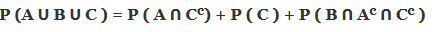A) TrueB) False

5) Consider a tetrahedral die and roll it twice. What is the probability that the number on the first roll is strictly higher than the number on the second roll?

Note: A tetrahedral die has only four sides (1, 2, 3 and 4).

A) 1/2
B) 3/8
C) 7/16
D) 9/16

6) Which of the following options cannot be the probability of any event?

A) -0.00001
B) 0.5
C) 1.001

A) Only A
B) Only B
C) Only C
D) A and B
E) B and C
F) A and C

Solution: (F)

Probability always lie within 0 to 1.

7) Anita randomly picks 4 cards from a deck of 52-cards and places them back into the deck ( Any set of 4 cards is equally likely ). Then, Babita randomly chooses 8 cards out of the same deck ( Any set of 8 cards is equally likely). Assume that the choice of 4 cards by Anita and the choice of 8 cards by Babita are independent. What is the probability that all 4 cards chosen by Anita are in the set of 8 cards chosen by Babita?

A)48C4 x 52C4

B)48C4 x 52C8

C)48C8 x 52C8

D) None of the above

Solution: (A)

The total number of possible combination would be 52C4 (For selecting 4 cards by Anita) * 52C8 (For selecting 8 cards by Babita).

Since, the 4 cards that Anita chooses is among the 8 cards which Babita has chosen, thus the number of combinations possible is 52C4 (For selecting the 4 cards selected by Anita) * 48C4 (For selecting any other 4 cards by Babita, since the 4 cards selected by Anita are common)

Question Context 8:

A player is randomly dealt a sequence of 13 cards from a deck of 52-cards. All sequences of 13 cards are equally likely. In an equivalent model, the cards are chosen and dealt one at a time. When choosing a card, the dealer is equally likely to pick any of the cards that remain in the deck.

8) If you dealt 13 cards, what is the probability that the 13th card is a King?

A) 1/52

B) 1/13

C) 1/26

D) 1/12

Solution: (B)

Since we are not told anything about the first 12 cards that are dealt, the probability that the 13th card dealt is a King, is the same as the probability that the first card dealt, or in fact any particular card dealt is a King, and this equals: 4/52

9) A fair six-sided die is rolled 6 times. What is the probability of getting all outcomes as unique?

A) 0.01543
B) 0.01993
C) 0.23148
D) 0.03333

Solution: (A)

For all the outcomes to be unique, we have 6 choices for the first turn, 5 for the second turn, 4 for the third turn and so on

Therefore the probability if getting all unique outcomes will be equal to 0.01543

10) A group of 60 students is randomly split into 3 classes of equal size. All partitions are equally likely. Jack and Jill are two students belonging to that group. What is the probability that Jack and Jill will end up in the same class?

A) 1/3
B) 19/59
C) 18/58
D) 1/2

Solution: (B)

Assign a different number to each student from 1 to 60. Numbers 1 to 20 go in group 1, 21 to 40 go to group 2, 41 to 60 go to group 3.

All possible partitions are obtained with equal probability by a random assignment if these numbers, it doesn’t matter with which students we start, so we are free to start by assigning a random number to Jack and then we assign a random number to Jill. After Jack has been assigned a random number there are 59 random numbers available for Jill and 19 of these will put her in the same group as Jack. Therefore the probability is 19/59

11) We have two coins, A and B. For each toss of coin A, the probability of getting head is 1/2 and for each toss of coin B, the probability of getting Heads is 1/3. All tosses of the same coin are independent. We select a coin at random and toss it till we get a head. The probability of selecting coin A is ¼ and coin B is 3/4. What is the expected number of tosses to get the first heads?

A) 2.75
B) 3.35
C) 4.13
D) 5.33

Solution: (A)

If coin A is selected then the number of times the coin would be tossed for a guaranteed Heads is 2, similarly, for coin B it is 3. Thus the number of times would be

Tosses = 2 * (1/4)[probability of selecting coin A] + 3*(3/4)[probability of selecting coin B]

= 2.75

12) Suppose a life insurance company sells a \$240,000 one year term life insurance policy to a 25-year old female for \$210. The probability that the female survives the year is .999592. Find the expected value of this policy for the insurance company.

A) \$131

B) \$140

C) \$112

D) \$125

Solution: (C)

P(company loses the money ) = 0.99592

P(company does not lose the money ) = 0.000408

The amount of money company loses if it loses = 240,000 – 210 = 239790

While the money it gains is \$210

Expected money the company will have to give = 239790*0.000408 = 97.8

Expect money company gets = 210.

Therefore the value = 210 – 98 = \$112

13)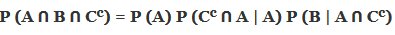A) TrueB) False

Solution: (A)

The above statement is true. You would need to know that

P(A/B) = P(AꓵB)/P(B)

P(CcꓵA|A) = P(CcꓵAꓵA)/P(A) = P(CcꓵA)/P(A)

P(B|A ꓵ Cc) = P(AꓵBꓵCc)/P(A ꓵ Cc)

Multiplying the three we would get – P(AꓵBꓵCc), hence the equations holds true

14) When an event A independent of itself?

A) Always
B) If and only if P(A)=0
C) If and only if P(A)=1
D) If and only if P(A)=0 or 1

Solution: (D)

The event can only be independent of itself when either there is no chance of it happening or when it is certain to happen. Event A and B is independent when P(AꓵB) = P(A)*P(B). Now if B=A, P(AꓵA) = P(A) when P(A) = 0 or 1.

15) Suppose you’re in the final round of “Let’s make a deal” game show and you are supposed to choose from three doors – 1, 2 & 3. One of the three doors has a car behind it and other two doors have goats. Let’s say you choose Door 1 and the host opens Door 3 which has a goat behind it. To assure the probability of your win, which of the following options would you choose.

C) It doesn’t matter probability of winning or losing is the same with or without revealing one door

Solution: (A)

16) Cross-fertilizing a red and a white flower produces red flowers 25% of the time. Now we cross-fertilize five pairs of red and white flowers and produce five offspring. What is the probability that there are no red flower plants in the five offspring?

A) 23.7%
B) 37.2%
C) 22.5%
D) 27.3%

Solution: (A)

The probability of offspring being Red is 0.25, thus the probability of the offspring not being red is 0.75. Since all the pairs are independent of each other, the probability that all the offsprings are not red would be (0.75)5 = 0.237. You can think of this as a binomial with all failures.

17) A roulette wheel has 38 slots – 18 red, 18 black, and 2 green. You play five games and always bet on red slots. How many games can you expect to win?

A) 1.1165

B) 2.3684C) 2.6316

C) 2.6316

D) 4.7368

Solution: (B)

The probability that it would be Red in any spin is 18/38. Now, you are playing the game 5 times and all the games are independent of each other. Thus, the number of games that you can win would be 5*(18/38) = 2.3684

18) A roulette wheel has 38 slots, 18 are red, 18 are black, and 2 are green. You play five games and always bet on red. What is the probability that you win all the 5 games?

A) 0.0368
B) 0.0238
C) 0.0526
D) 0.0473

Solution: (B)

The probability that it would be Red in any spin is 18/38. Now, you are playing for game 5 times and all the games are independent of each other. Thus, the probability that you win all the games is (18/38)5 = 0.0238

19) Some test scores follow a normal distribution with a mean of 18 and a standard deviation of 6. What proportion of test takers have scored between 18 and 24?

A) 20%
B) 22%
C) 34%
D) None of the above

Solution: (C)

So here we would need to calculate the Z scores for value being 18 and 24. We can easily doing that by putting sample mean as 18 and population mean as 18 with σ = 6 and calculating Z. Similarly we can calculate Z for sample mean as 24.

Z= (X-μ)/σ

Therefore for 26 as X,

Z = (18-18)/6  = 0 , looking at the Z table we find 50% people have scores below 18.

For 24 as X

Z = (24-18)/6  = 1, looking at the Z table we find 84% people have scores below 24.

Therefore around 34% people have scores between 18 and 24.

20) A jar contains 4 marbles. 3 Red & 1 white. Two marbles are drawn with replacement after each draw. What is the probability that the same color marble is drawn twice?

A) 1/2
B) 1/3
C) 5/8
D) 1/8

Solution: (C)

If the marbles are of the same color then it will be 3/4 * 3/4 + 1/4 * 1/4 = 5/8.

21) Which of the following events is most likely?

A) At least one 6, when 6 dice are rolledB) At least 2 sixes when 12 dice are rolled

C) At least 3 sixes when 18 dice are rolled

D) All the above have same probability

Solution: (A)

Probability of ‘6’ turning up in a roll of dice is P(6) = (1/6) & P(6’) = (5/6). Thus, probability of

Case 1: (1/6) * (5/6)5 = 0.06698

Case 2: (1/6)2 * (5/6)10 = 0.00448

Case 3: (1/6)3 * (5/6)15 = 0.0003

Thus, the highest probability is Case 1

22) Suppose you were interviewed for a technical role. 50% of the people who sat for the first interview received the call for second interview. 95% of the people who got a call for second interview felt good about their first interview. 75% of people who did not receive a second call, also felt good about their first interview. If you felt good after your first interview, what is the probability that you will receive a second interview call?

A) 66%

B) 56%

C) 75%

D) 85%

Solution: (B)

Let’s assume there are 100 people that gave the first round of interview. The 50 people got the interview call for the second round. Out of this 95 % felt good about their interview, which is 47.5. 50 people did not get a call for the interview; out of which 75% felt good about, which is 37.5. Thus, the total number of people that felt good after giving their interview is (37.5 + 47.5) 85. Thus, out of 85 people who felt good, only 47.5 got the call for next round. Hence, the probability of success is (47.5/85) = 0.558.

Another more accepted way to solve this problem is the Baye’s theorem. I leave it to you to check for yourself.

23) A coin of diameter 1-inches is thrown on a table covered with a grid of lines each two inches apart. What is the probability that the coin lands inside a square without touching any of the lines of the grid? You can assume that the person throwing has no skill in throwing the coin and is throwing it randomly.

You can assume that the person throwing has no skill in throwing the coin and is throwing it randomly.

A) 1/2
B) 1/4
C) Π/3
D) 1/3

Solution: (B)

Think about where all the center of the coin can be when it lands on 2 inches grid and it not touching the lines of the grid.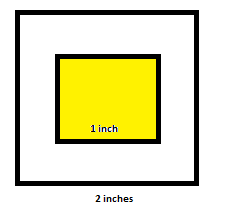If the yellow region is a 1 inch square and the outside square is of 2 inches. If the center falls in the yellow region, the coin will not touch the grid line. Since the total area is 4 and the area of the yellow region is 1, the probability is ¼ .

24) There are a total of 8 bows of 2 each of green, yellow, orange & red. In how many ways can you select 1 bow?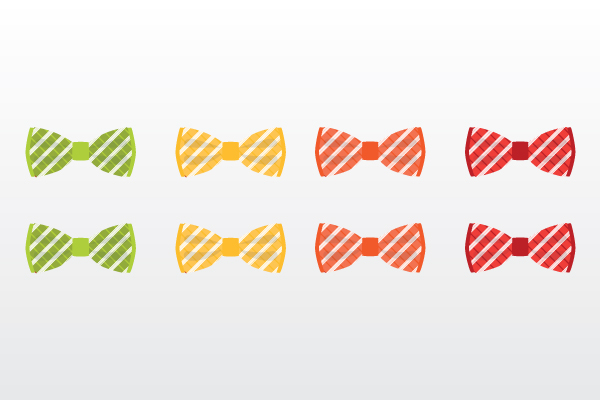A) 1
B) 2
C) 4
D) 8

Solution: (C)

You can select one bow out of four different bows, so you can select one bow in four different ways.

25) Consider the following probability density function: What is the probability for X≤6 i.e. P(x≤6)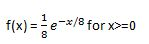What is the probability for X≤6 i.e. P(x≤6)

A) 0.3935
B) 0.5276
C) 0.1341
D) 0.4724

Solution: (B)

To calculate the area of a particular region of a probability density function, we need to integrate the function under the bounds of the values for which we need to calculate the probability.

Therefore on integrating the given function from 0 to 6, we get 0.5276

26) In a class of 30 students, approximately what is the probability that two of the students have their birthday on the same day (defined by same day and month) (assuming it’s not a leap year)?

For example – Students with birthday 3rd Jan 1993 and 3rd Jan 1994 would be a favorable event.

A) 49%

B) 52%

C) 70%

D) 35%

Solution: (C)

The total number of combinations possible for no two persons to have the same birthday in a class of 30 is 30 * (30-1)/2 = 435.

Now, there are 365 days in a year (assuming it’s not a leap year). Thus, the probability of people having a different birthday would be 364/365. Now there are 870 combinations possible. Thus, the probability that no two people have the same birthday is (364/365)^435 = 0.303.

Thus, the probability that two people would have their birthdays on the same date would be 1 – 0.303 = 0.696

27) Ahmed is playing a lottery game where he must pick 2 numbers from 0 to 9 followed by an English alphabet (from 26-letters). He may choose the same number both times.

If his ticket matches the 2 numbers and 1 letter drawn in order, he wins the grand prize and receives \$10405. If just his letter matches but one or both of the numbers do not match, he wins \$100. Under any other circumstance, he wins nothing. The game costs him \$5 to play. Suppose he has chosen 04R to play.

What is the expected net profit from playing this ticket?

A) \$-2.81

B) \$2.81C) \$-1.82

C) \$-1.82

D) \$1.82

Solution: (B)

Expected value in this case

E(X) = P(grand prize)*(10405-5)+P(small)(100-5)+P(losing)*(-5)

P(grand prize)=  (1/10)*(1/10)*(1/26)

P(small) = 1/26-1/2600, the reason we need to do this is we need to exclude the case where he gets the letter right and also the numbers rights. Hence, we need to remove the scenario of getting the letter right.

P(losing ) = 1-1/26-1/2600

Therefore we can fit in the values to get the expected value as \$2.81

28) Assume you sell sandwiches. 70% people choose egg, and the rest choose chicken. What is the probability of selling 2 egg sandwiches to the next 3 customers?

A) 0.343
B) 0.063
C) 0.44
D) 0.027

Solution: (C)

The probability of selling Egg sandwich is 0.7 & that of a chicken sandwich is 0.3. Now, the probability that next 3 customers would order 2 egg sandwich is 3 * 0.7 * 0.7 *0.3 = 0.44. They can order them in any sequence, the probabilities would still be the same.

Question context: 29 – 30

HIV is still a very scary disease to even get tested for. The US military tests its recruits for HIV when they are recruited. They are tested on three rounds of Elisa( an HIV test) before they are termed to be positive.

The prior probability of anyone having HIV is 0.00148. The true positive rate for Elisa is 93% and the true negative rate is 99%.

29) What is the probability that a recruit has HIV, given he tested positive on first Elisa test? The prior probability of anyone having HIV is 0.00148. The true positive rate for Elisa is 93% and the true negative rate is 99%.

A) 12%
B) 80%
C) 42%
D) 14%

Solution: (A)

I recommend going through the Bayes updating section of this article for the understanding of the above question.

30) What is the probability of having HIV, given he tested positive on Elisa the second time as well.

The prior probability of anyone having HIV is 0.00148. The true positive rate for Elisa is 93% and the true negative rate is 99%.

A) 20%
B) 42%
C) 93%
D) 88%

Solution: (C)

I recommend going through the Bayes updating section of this article for the understanding of the above question.

31) Suppose you’re playing a game in which we toss a fair coin multiple times. You have already lost thrice where you guessed heads but a tails appeared. Which of the below statements would be correct in this case?

A) You should guess heads again since the tails has already occurred thrice and its more likely for heads to occur now
B) You should say tails because guessing heads is not making you win
C) You have the same probability of winning in guessing either, hence whatever you guess there is just a 50-50 chance of winning or losing
D) None of these

Solution: (C)

This is a classic problem of gambler’s fallacy/monte carlo fallacy, where the person falsely starts to think that the results should even out in a few turns. The gambler starts to believe that if we have received 3 heads, you should receive a 3 tails. This is however not true. The results would even out only in infinite number of trials.

32) The inference using the frequentist approach will always yield the same result as the Bayesian approach.

A) TRUE
B) FALSE

Solution: (B)

The frequentist Approach is highly dependent on how we define the hypothesis while Bayesian approach helps us update our prior beliefs. Therefore the frequentist approach might result in an opposite inference if we declare the hypothesis differently. Hence the two approaches might not yield the same results.

33) Hospital records show that 75% of patients suffering from a disease die due to that disease. What is the probability that 4 out of the 6 randomly selected patients recover?

A) 0.17798
B) 0.13184
C) 0.03295
D) 0.35596

Solution: (C)

Think of this as a binomial since there are only 2 outcomes, either the patient dies or he survives.

Here n =6, and x=4.  p=0.25(probability if living(success)) q = 0.75(probability of dying(failure))

P(X) = nCx pxqn-x = 6C4 (0.25)4(0.75)2 = 0.03295

34) The students of a particular class were given two tests for evaluation. Twenty-five percent of the class cleared both the tests and forty-five percent of the students were able to clear the first test.

Calculate the percentage of students who passed the second test given that they were also able to pass the first test.

A) 25%

B) 42%

C) 55%
D) 45%

Solution: (C)

This is a simple problem of conditional probability. Let A be the event of passing in first test.

B is the event of passing in the second test.

P(AꓵB) is passing in both the events

P(passing in second given he passed in the first one) = P(AꓵB)/P(A)

= 0.25/0.45 which is around 55%

35) While it is said that the probabilities of having a boy or a girl are the same, let’s assume that the actual probability of having a boy is slightly higher at 0.51. Suppose a couple plans to have 3 children. What is the probability that exactly 2 of them will be boys?

A) 0.38
B) 0.48
C) 0.58
D) 0.68
E) 0.78

Solution: (A)

Think of this as a binomial distribution where getting a success is a boy and failure is a girl. Therefore we need to calculate the probability of getting 2 out of three successes.

P(X) = nCx pxqn-x = 3C2 (0.51)2(0.49)1 = 0.382

36) Heights of 10 year-olds, regardless of gender, closely follow a normal distribution with mean 55 inches and standard deviation 6 inches. Which of the following is true?

A) We would expect more number of 10 year-olds to be shorter than 55 inches than the number of them who are taller than 55 inches
B) Roughly 95% of 10 year-olds are between 37 and 73 inches tall

C) A 10-year-old who is 65 inches tall would be considered more unusual than a 10-year-old who is 45 inches tall

D) None of these

Solution: (D)

None of the above statements are true.

37) About 30% of human twins are identical, and the rest are fraternal. Identical twins are necessarily the same sex, half are males and the other half are females. One-quarter of fraternal twins are both males, one-quarter both female, and one-half are mixed: one male, one female. You have just become a parent of twins and are told they are both girls. Given this information, what is the probability that they are identical?

A) 50%

B) 72%
C) 46%
D) 33%

Solution: (C)

This is a classic problem of Bayes theorem.

P(I) denoted Probability of being identical and P(~I) denotes Probability of not being identical

P(Identical) = 0.3

P(not Identical)= 0.7

P(FF|I)= 0.5

P(MM|I)= 0.5

P(MM|~I)= 0.25

P(FF|~I)= 0.25

P(FM|~I)= 0.25

P(I|FF) = 0.46

38) Rob has fever and the doctor suspects it to be typhoid. To be sure, the doctor wants to conduct the test. The test results positive when the patient actually has typhoid 80% of the time. The test gives positive when the patient does not have typhoid 10% of the time. If 1% of the population has typhoid, what is the probability that Rob has typhoid provided he tested positive?

A) 12%
B) 7%
C) 25%
D) 31.5%

Solution: (B)

We need to find the probability of having typhoid given he tested positive.

=P(testing +ve and having typhoid) / P(testing positive)

= = 0.074

39) Jack is having two coins in his hand. Out of the two coins, one is a real coin and the second one is a faulty one with Tails on both sides. He blindfolds himself to choose a random coin and tosses it in the air. The coin falls down with Tails facing upwards. What is the probability that this tail is shown by the faulty coin?

A) 1/3
B) 2/3
C) 1/2
D) 1/4

Solution: (B)

We need to find the probability of the coin being faulty given that it showed tails.

P(Faulty) = 0.5

P(getting tails) = 3/4

P(faulty and tails) =0.5*1 = 0.5

Therefore the probability of coin being faulty given that it showed tails would be 2/3

40) A fly has a life between 4-6 days. What is the probability that the fly will die at exactly 5 days?

A) 1/2

B) 1/4

C) 1/3
D) 0

Solution: (D)

Here since the probabilities are continuous, the probabilities form a mass function. The probability of a certain event is calculated by finding the area under the curve for the given conditions. Here since we’re trying to calculate the probability of the fly dying at exactly 5 days – the area under the curve would be 0. Also to come to think of it, the probability if dying at exactly 5 days is impossible for us to even figure out since we cannot measure with infinite precision if it was exactly 5 days.

## End Notes

If you missed out on this competition, make sure you complete in the ones coming up shortly. We are giving cash prizes worth \$10,000+ during the month of April 2017.

If you have any questions or doubts feel free to post them below.

### Check out all the upcoming skilltests here.###### Dishashree26 Gupta

Dishashree is passionate about statistics and is a machine learning enthusiast. She has an experience of 1.5 years of Market Research using R, advanced Excel, Azure ML.

## 41 thoughts on "40 Questions on Probability for data science"###### Kenneth Singh says:April 10, 2017 at 7:32 am
Hi, None of the options in question 7 are probability values right? I think all of them are Numerators. Shouldn't the correct option be "None of the above"? Reply###### Chen Luo says:April 10, 2017 at 12:51 pm
Maybe this problem should be 'the number of possible cases'. Reply###### Arcady Novosyolov says:April 10, 2017 at 1:25 pm
Hello, Thanks for publishing this set of problems. It looks like the answer to the question 21 is right (option A), but solution is wrong, since actual probabilities of events are P(A) = 0.402, P(B) = 0.296, and P(C) = 0.245, see explanation at https://github.com/arcadynovosyolov/math_and_prob/blob/master/note_on_question_21.ipynb Best regards, Arcady Reply###### santiago says:April 10, 2017 at 2:12 pm
About question 2, Why you do that? If you ask something with a real example please give a real answer. Do you really think that the probability is 0.33? The result is 0.5. The events are independant. Reply###### Dishashree Gupta says:April 10, 2017 at 2:31 pm
This is a problem of conditional probability and we have defined the case where one child is already a girl. Now the sample set reduces and hence we have only 3 choices in the sample set. Reply###### santiago says:April 10, 2017 at 2:40 pm
I understand the idea, but if your objective is to test conditional probability maybe another example could be better. In real life the sex of one of your childs doesn't affect the sex of those who will come Reply###### Oshan says:April 10, 2017 at 2:58 pm
for Q 21. The cases considered are for exactly 1, 2 and 3 sixes respectively. however, the question states "atleast" 1,2 and 3 sixes. I am not sure if this would make a difference in the final answer, but this seems like a mistake. Kindly clarify in case I am missing something here.. Reply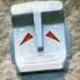###### Andrew Morris says:April 10, 2017 at 5:12 pm
The answer to question 7 must be wrong, because it is greater than 1. The English grammar through these questions also has many mistakes, e.g. "are common" should be "are in common". Reply###### [email protected]says:April 10, 2017 at 8:47 pm
Hi! Could you please clarify the following: Q7 - The answer A) can not be the correct one since it is > 1 (see Q6 :) ). So, D.) should be the right choice Q28 - it is the Binomial schema, isn't it? Probability of success (p) = 0.7, q = 0.3, n = 3 and m = 2. Then, answer is 3 * 0.7^2 * 0.3 = 0.44. The choice C.) is incorrect since 0.7*0.3*0.7 and 0.3*0.7*0.7 have to be considered as well. Thanks. Reply###### [email protected]says:April 10, 2017 at 9:33 pm
Also, regarding the Q2 - it is well-known "Boy or Girl paradox" (https://en.wikipedia.org/wiki/Boy_or_Girl_paradox). And both answers - C.) and A.) - are correct depending on the randomizing procedure. Reply###### Aditya Royal Matturi says:April 11, 2017 at 5:16 am
The answer for question 7 is (1/52 c 4)*(48 c 4/52 c 4) please correct that Reply###### Aditya Royal Matturi says:April 11, 2017 at 5:17 am
The answer for question 7 is (1/52 c 4)*(48 c 4/52 c 4) please correct that Reply###### Aditya Royal Matturi says:April 11, 2017 at 7:33 am
As we are concerned about statistics here it definitely effects statistically, I am also sure that it doesn't effect genetically and it's pure luck and as always luck as has some role in many things like tossing coins etc but we are ignorant of it. Reply###### Soham Lawar says:April 11, 2017 at 7:53 am
Hello , I have following queries ; 1) I request you to elaborate more for question 9 2) In question 19 range of test scores is 18 to 24 and in the explanation you have explained for range 20 to 26 3) In the explanation of question number 40 I think 'since the probabilities are continuous, the probabilities form a distribution function ' is correct Regards, Soham Reply###### potterhead says:April 11, 2017 at 8:18 am
The answer for question 7 should be D - None of these. None of the options is a probability value. Reply###### Santhosh says:April 11, 2017 at 10:48 am
Q28 & Q33 both looks similar to me as both are having binomial outputs . Could you please explain why is it different for Q27 and why cant we use Binomial distribution for this unlike Q33 Reply###### Aditya Royal Matturi says:April 11, 2017 at 10:50 am
question 23 is invalid because there can be an infinite number of ways of throwing a coin, though to satisfy the condition there is only one possible way, there are infinite total outcomes for that test case so I guess1/infinite=~0 Reply###### Aditya Royal Matturi says:April 11, 2017 at 10:54 am###### [email protected]says:April 11, 2017 at 11:57 am
Hi! Could you please clarify the following: Q7 – The answer A) can not be the correct one since it is > 1 (see Q6 ? ). So, D.) should be the right choice Q28 – it is the Binomial schema, isn’t it? Probability of success (p) = 0.7, q = 0.3, n = 3 and m = 2. Then, answer is 3 * 0.7^2 * 0.3 = 0.44. The choice C.) is incorrect since 0.7*0.3*0.7 and 0.3*0.7*0.7 have to be considered as well. Thanks. Reply###### hanna yang says:April 11, 2017 at 12:49 pm
i think the explanation of question 4 "P(AꓵCc) will be only P(A)."is wrong. it shoud be P(AꓵCc) will be P(A-C), If AꓵC =Φ,then the explanation"P(AꓵCc) will be only P(A)."must be right.. Reply###### Stu says:April 11, 2017 at 4:42 pm
I believe the correct answer to 7 is B not A. Your reasoning is correct, but I think you made an error in reducing the fraction. Reply###### M Zakaria says:April 12, 2017 at 8:11 am
Regarding Q4. I think we should state explicitly that A, B, and C are independent. This way P(AUBUC) = P(A) + P(B) + P(C). otherwise we need to subtract P(A and B), P(A and C), and P(B and C) from the result. Reply###### Mustafa V says:April 12, 2017 at 9:46 am###### Mustafa V says:April 12, 2017 at 2:16 pm
Q2. Answer should be 0.5. Can be reasoned in 2 ways. 1st child & 2nd child are independent events and it is mentioned that population is balanced, p=.5. Also since 1st child is girl so of the possibilities only gb and gg valid, again p = 0.5. Reply###### David Harper says:April 13, 2017 at 5:19 pm
Hi Dishashree, The flaw is that {BB, GG, BG, GB} represent a permutation. When you reveal that the first is a girl, you are revealing GX or XG and excluding BB and BX so that 2/4 remain. Put another way, there are 3 combinations {BB, BG or GB, and GG} such that revealing one girl eliminates BB and what remains is 1/2. I hope that's helpful! Reply###### Mustafa V says:April 14, 2017 at 5:53 am
Hi, Regarding Q10 - the solution I have is as follows:- Jack & Jill in one section - number of ways is (58C18)*(40C20) [A] Total number of ways is (60C20)*(40C20) [B] Thus the required probability is A/B which is 19/177. But this option is not there at all. Can you review my solution? Reply###### 40 Questions on Probability for data science says:April 16, 2017 at 1:01 am###### Nimrod Ifrach says:May 12, 2017 at 7:24 am
Hi, Im not sure the answer to q28 is correct. 0.147 is the probability of only 1 case that can happen, but there are 3 possible cases: 1. Customers 1+2 buys egg 2. Customers 1+3 buys egg 3. Customers 2+3 buys egg So actually its 0.147*3=0.44 Reply###### Dishashree Gupta says:May 12, 2017 at 1:39 pm
Both Q7 and 28 were removed from the final score calculation due to discrepancy ! Reply###### Dishashree Gupta says:May 12, 2017 at 1:43 pm
Can you give more detail on your A and B calculation ? Reply###### Dishashree Gupta says:May 12, 2017 at 1:44 pm
Yes there was an issue with it. It had been removed from the final score calculation Reply###### Dishashree Gupta says:May 12, 2017 at 1:44 pm
Yes, it has been removed from the final score calculation Reply###### Dishashree Gupta says:May 12, 2017 at 1:46 pm
Yes, the answer for Q7 is not mentioned. We have removed it from the final calculation. Reply###### Dishashree Gupta says:May 12, 2017 at 1:48 pm
Yes, we have removed 7 from score calculation. Reply###### Dishashree Gupta says:May 12, 2017 at 2:18 pm
1) Elaborating on Q9, what I wanted you to calculate is the probability that when you throw a dice 6 times, you should get 1,2,3,4,5,6 in some order. 2) Q19 - this has been rectified. 3) yes, so in case of a distribution function, the probability of a random variable being exactly equal to a particular value is 0. We can only calculate the probability of a random variable being in a range. Reply###### Dishashree Gupta says:May 24, 2017 at 4:39 pm
We removed 7 from the calculation due to discrepancy ! Reply###### Sam Gu says:June 02, 2017 at 5:27 pm
There are 2 variants of this question: 1. Alice has 2 kids and the elder one is a girl. What is the probability that the younger child is also a girl? The answer is 50%. 2. Alice has 2 kids and one of them is a girl. What is the probability that the other child is also a girl? The answer is 33%, as explained in the answer key. Reply###### Sarah Nogueira says:October 30, 2017 at 7:24 pm
For Q11, the solution says: "If coin A is selected then the number of times the coin would be tossed for a guaranteed Heads is 2". This does not seem right since the probability of getting heads with coin A is 1/2, and each toss is independent, we could toss coin A a hundred times and never observe heads... Reply###### Dishashree Gupta says:October 30, 2017 at 7:36 pm
Hi Sarah, If the probability of getting a heads from a coin is 1/2, can you really say that you would get a heads if you throw the coin twice ? Reply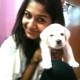###### MJ says:April 10, 2018 at 1:44 pm
No we can't, thats why I think the solution is flawed. Reply